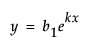Publication date: 05/24/2021

## Customize the Nonlinear Model Library

The Model Library is created by a built-in script named NonlinLib.jsl, located in the Resources/Builtins folder in the folder that contains JMP (Windows) or in the Application Package (macOS). You can customize the nonlinear library script by modifying this script.

Note: You might not be able to directly edit the file. If this is the case, open the script, make the desired edits, and save to a different location on your computer. Use the same filename. Then, delete the old NonlinLib.jsl script in the Resources/Builtins folder and move the new NonlinLib.jsl file into the Resources/Builtins folder.

To add a model, you must add three lines to the list named Listofmodellist#. These three lines are actually a list themselves, which consists of the following three parts.

Model name, a quoted string

Model formula, an expression

Model scale

For example, suppose you want to add a model called “Simple Exponential Growth” that has the formAdd the following lines to the NonlinLib.jsl script

`{//Simple Exponential Growth`
`		"Simple Exponential Growth",`
`		Expr(Parameter({b1=2, k=0.5}, b1*exp(k * :X))),`
`		lowx = -1; highx = 2; lowy = 0;  highy = 2},`

Some things to note:

The first line is simply an open bracket (starting the list) and an optional comment. The second line is the string that is displayed in the model library window.

The values of lowx, highx, logy, and highy specify the initial window for the theoretical graph.

There is a comma as the last character in the example above. If this is the final entry in the Listofmodellist# list, the comma can be omitted.

If the model uses more than two parameters, replace the last line (containing the graph limits) with the quoted string “String Not Available”.

To delete a model, delete the corresponding three-lined list from the Listofmodellist# list.